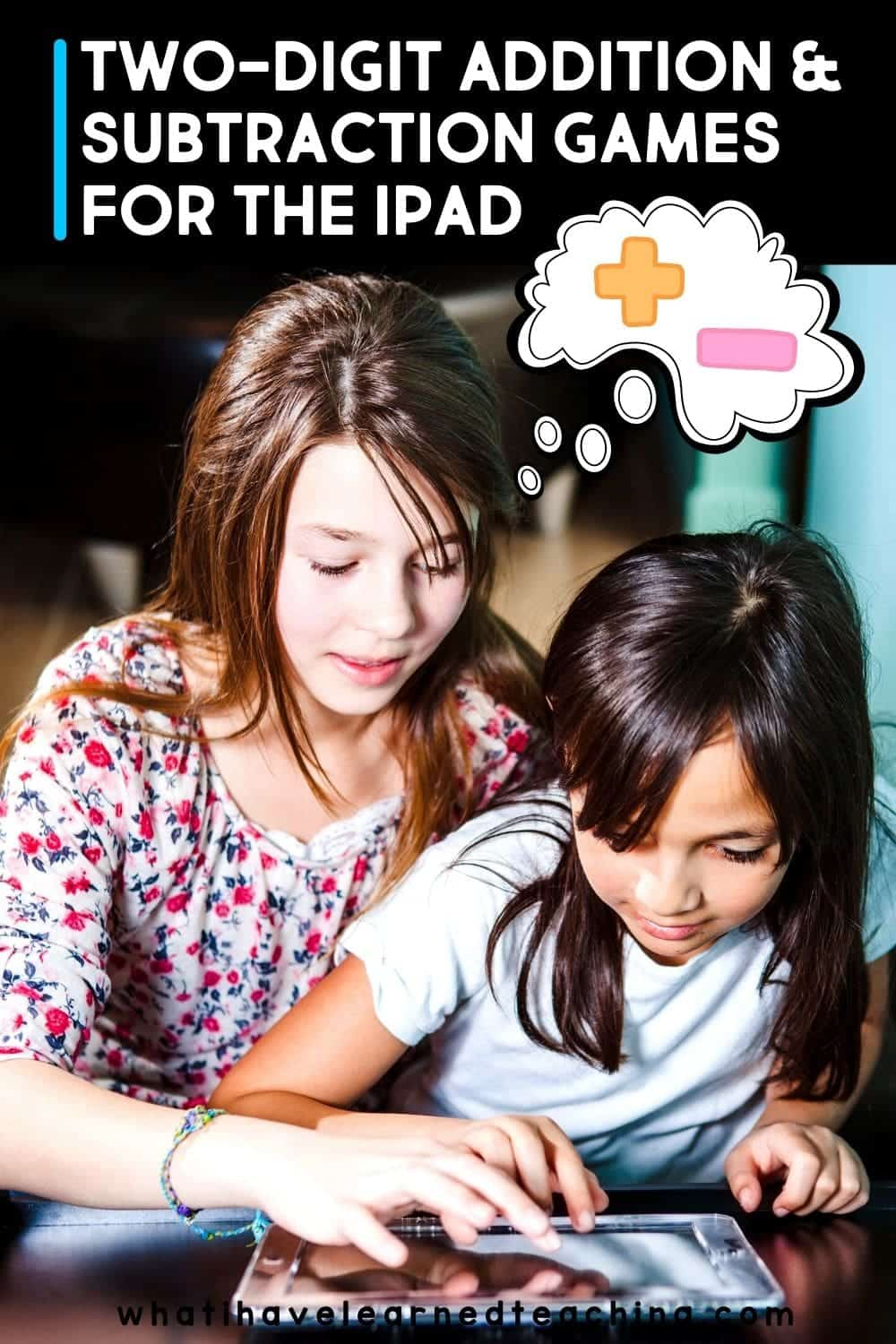There are many different strategies to teach two-digit addition and subtraction. In line with standards, you’ve probably focused on place value, properties of operations, and relationship between addition and subtraction. You may also show different models such as number lines or base-10 blocks. To practice these strategies and the models associated with them, try integrating technology in your lessons. Here are the 6 best iPad apps for two-digit addition and subtraction.

A Math Regrouping App: Addition and Subtraction HD

This app allows students to solve problems just as they would on paper using their finger or a stylus to write out the steps and regrouping. Cute animals help students learn. Options for numbers of questions and time are available.

Math Evolve

Two modes allow students to learn and explore different math concepts and skills including two-digit addition and subtraction. Try story mode where students will go on a math adventure while practicing math facts. Practice mode works as an alternative to flashcards for mental math and memorization. Story mode is your best bet in practicing the skills needed for two-digit problem solving.

Math Wise

This app not only gives your students a chance to practice their addition and subtraction, it also allows you to view analytics on their progress and input your whole class.

Teaching Number Lines

Use a digital format for teaching number lines, one of the tried and true models for teaching two-digit addition and subtraction. The app uses little frogs to show another way of completing addition and subtraction problems.

This award winning app from the Splash Math series has 71+ grade 2 math skills and 350 targeted at K-5. When working with two-digit addition and subtraction, this app allows students to work at their own pace, have a scratch pad for work space, and gives explanations for incorrect answers.

Math Slide

Okay – this one it’s about two-digit addition and subtraction, but it has apps for all the prerequisite skills to do two-digit addition and subtraction. It’s a fun partner game where students solve whatever is in the center and push the answer to the center. The first one to solve it wins. There are five different apps that include games for tens and ones, addition and subtraction facts, multiplication and division facts, hundreds, tens and ones, and basic facts. All of these skills can be used to develop strategies to add and subtraction multi-digit numbers.

These apps are additional tools to add to your toolbox when teaching and practicing two-digit addition and subtraction. Your students will enjoy the games and animations while you’ll love seeing their skills and independence improve. Try out these 6 best iPad apps for two-digit addition and subtraction to supplement your lessons.

## Check out these Two-Digit Addition & Subtraction Blog Posts